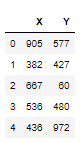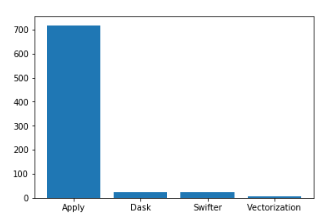# Parallelize pandas apply using dask and swifter

Using Pandas apply function to run a method along all the rows of a dataframe is slow and if you have a huge data to apply thru a CPU intensive function then it may take several seconds also.

In this post we are going to explore how we can partition the dataframe and apply the functions on this partitions using dask and other library and methods for parallel processing like swifter & Vectorization respectively

## What is Parallel processing?

Parallel computing is a task where a large chunk of data is divided into smaller parts and processed simultaneously using the modern hardware capability of multiple CPU’s and cores of the machine.

## Why Pandas apply function is slow?

The apply function does not take advantage of Vectorization and it returns a new Series and Dataframe objects and that’s the reason with a large dataset running with a for loop under the hood and overhead of IO operations makes it slow

Dask is a library for parallel computing in Python and it is basically used for the following two tasks:

a) Task Scheduler: It is used for optimizing the task scheduling jobs just like celery, Luigi etc.

b) Store the data in Parallel Arrays, Dataframe and it runs on top of task scheduler

• Familiar: Provides parallelized NumPy array and Pandas DataFrame objects
• Flexible: Provides a task scheduling interface for more custom workloads and integration with other projects.
• Native: Enables distributed computing in pure Python with access to the PyData stack.
• Fast: Operates with low overhead, low latency, and minimal serialization necessary for fast numerical algorithms
• Scales up: Runs resiliently on clusters with 1000s of cores
• Scales down: Trivial to set up and run on a laptop in a single process
• Responsive: Designed with interactive computing in mind, it provides rapid feedback and diagnostics to aid humans

## Create a Big Dataframe of 100K rows

Build a dataframe with 100K rows and two columns with values selected randomly between 1 and 1000

``````import pandas as pd
import numpy as np
df = pd.DataFrame({'X':np.random.randint(1000, size=100000),'Y':np.random.randint(1000, size=100000)})
``````## Function to add the sum of squares of each column

This function will take a dataframe object and will return the sum of square for each column

``````def add_squares(df):
return df.x**2+df.y**2
``````

## timeit the `add_squares` function on dataframe

We will apply the `add_squares` functions on the dataframe and timeit the execution to see how long it takes to calculate the sum of square of columns for each row

``````%%timeit
``````

7.18 s ± 699 ms per loop (mean ± std. dev. of 7 runs, 1 loop each)

It took average around 7.18 secs per loop to apply `add_squares` method on this dataframe of 100K rows and 2 columns

Let’s see if we can speed it up using the Dask

In this section we are going to see how to make partitions of this big dataframe and parallelize the `add_squares` method

We will construct a dask dataframe from pandas dataframe using `from_pandas` function and specify the number of partitions(nparitions) to break this dataframe into

We will break into 4 parition since my windows laptop is configured with a 4 core and 16GB memory/

``````import dask.dataframe as dd
ddf = dd.from_pandas(df, npartitions=4)
``````

Next we will apply `add_squares` method on each of these partitions

``````%%timeit
``````

24.3 ms ± 6.91 ms per loop (mean ± std. dev. of 7 runs, 10 loops each)

We will use timeit to capture the time it takes to apply the `add_sqaure` method on each parition

compute() at the end will load the data into memory

## How fast is dask map_partition?

So we have seen dask `map_paritions` apply the `add_squares` method on this big dataframe in 24.3ms which is like 300X faster than the pandas apply function

## Swifter

You can check their documentation to see how to install using conda and pip

As per their documentation it defines Swifter as:

A package which efficiently applies any function to a pandas dataframe or series in the fastest available manner

``````def add_squares(a,b):
return a**2+b**2
``````

We have added a new `add_squares` function which takes two arguments and returns their sum of squares

``````%%timeit
``````

23.4 ms ± 3.71 ms per loop (mean ± std. dev. of 7 runs, 1 loop each)

## Vectorization

As far as possible you should try to vectorize the function and other options like iterrows and looping should be the last resort. Here we will see how can you vectorize the add_squares method

``````%%timeit
``````

5.56 ms ± 980 µs per loop (mean ± std. dev. of 7 runs, 100 loops each)

## Compare the speed of all methods

We have used four different ways to apply the add_squares method to our 100K dataframe rows

a) Pandas apply

b) Dask `map_partition`

c) Swifter

d) Vectorization

We will plot all the four timings in a bar graph## Conclusion

It is evident from the above result that Vectorization is a clear winner here which takes the minimum time to apply the add_squares method along the rows of the dataframe.

Dask Map_Partition and Swifter almost takes the same time to apply this method and compute the result for all the rows

So our first choice should be Vectorization and Just in case you are not able to Vectorize your function then you can use Dask map_parition and Swifter by paritioning te dataframe into multiple paritions and then running the function parallely on all these paritions

If you know any other methods or library which can parallelize the computation or run it parallely in Pandas then share it in the comments section below

Tags:

Categories:

Updated: